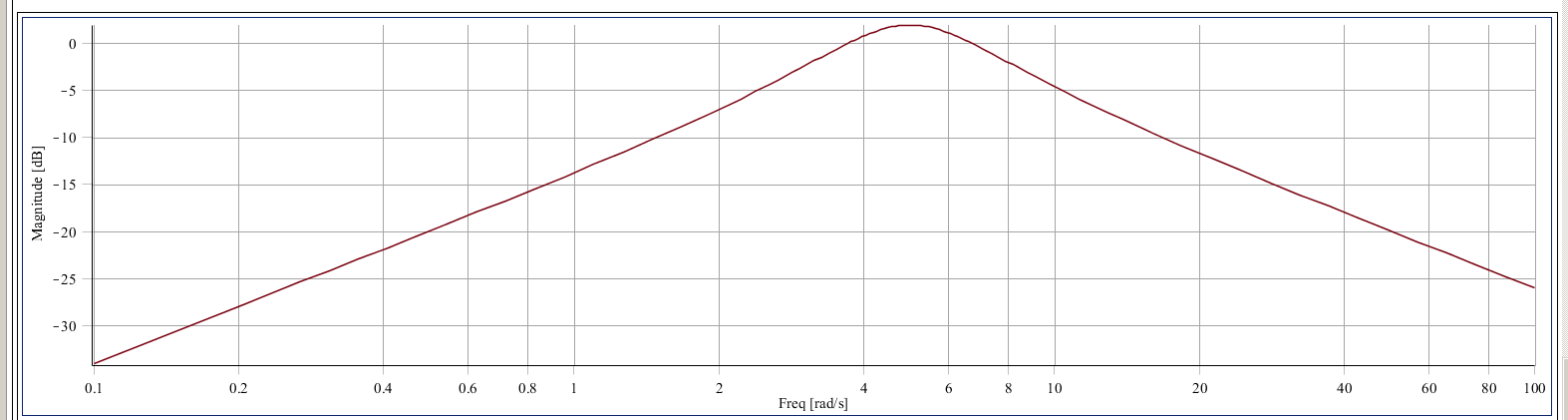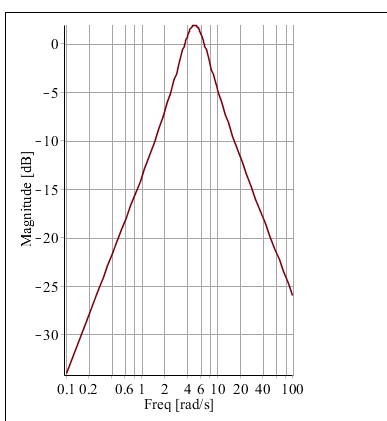## Print arguments of a function(procedure)...

Hi,

This maybe a really simple question, I remember this is doable but couldnt find where the answer is.

Say I write a function to sum up all values in the list,

mysum:=proc(data::list)

return printf("The sum is %3.3f. ", sum(data[i],i=1..numelems(data)));

end proc;

and I use it like,

mysum(listA);

mysum(listB);

"The sum of listA is %3.3f. "

"The sum of listB is %3.3f. "

Use "data", the input, as a string argument.

Thanks,

casper

## Writing symbols and equations...

I want to write an equation in the text portion of my document.  I do not need it evaluated. I just want to write formulas, like I do in LaTeX.

## How do I classify elements into sets in Maple?...

Hello!

I would like to start with the following set of 9 elements,
A = { E11, E12, E21, E22, E11+E12, E11+E21, E12+E22, E21+E22, E11+E12+E21+E22 }.

I need a procedure that takes each of those elements and creates 3 new ones in the following way: Eij becomes Eij1, Eij2, Eij1+Eij2. So for example, E11 will become: E111, E112, and E111+E112. And for example the fifth element in A (i.e. E11+E12) will become the 3 new elements: E111+E121, E112+E122, and E111+E121 + E112+E122.

Since each of the 9 elements gets triplicated, there will be a new set, call it B, with 27 elements.

B = {E111, E112, E111+E112, E121, E122, E121+E122, ... }

Now I want to repeat this process of triplicating again so that, for example, E111 becomes: E1111, E1112, and E1111+E1112. And so on. This new set C will have 81 elements. Now I want to repeat this one last time. The final set, D, will have 243 (3^5) elements.

Step 2:

For every pair of elements x and y in D, I want to compute z:=(x+y)mod2. If z already belongs to D, discard it, otherwise, place z in the set D2. Do this until there are no more elements to add together (note that if x+y is computed then I don't want y+x to be computed also--that's inefficient). Maybe the most efficient way is to perform all possibly combinations of x+y mod 2 to create the set D2 and then just go: D2 setminus D.

Step 3: For x in D and y in D2 perform all possible combinations of z:=(x+y)mod2 and place these in D3 then perform set subtraction again: D3 minus D2 minus D.

Repeat this process again: x in D and y in D3 to create new elements in D4. Repeat again until Dm is empty (that is, D(m-1) will be the last set that contains new elements). I'm expecting around 12 sets...

The issue with this whole algorithm is that I often run out of memory so I need a clever way to do this, since this algorithm is essentially classifying 2^32 elements into disjoint sets. Thank you!

## execution time difference between seq and \$ syntax...

When the loop variable can be written as a unit step sequence, I never really distinguish between using

seq( f(i), i=m..n ), and

f(i) \$ i=m..n

However I recent came across a case where the 'seq' construct ran about 2.5x faster. Is using 'seq' always faster? Does it depend on the function being evaluated? Why is there such a large difference in execution time

The original example which exhibited the problem is shown below, although after some experimentation, I have found other cases where 'seq' is faster (and plenty where it doesn't seem to make any difference!)

Example code for implementation using '\$' is

restart:
ulim:=1000000:
t1:=time():
ans:= max
( { iquo(3*d, 7)/d \$ d = 1..ulim }
minus
{3/7}
):
t2:= time()-t1;

Example code for for implementation using 'seq' is

restart:
ulim:=1000000:
t1:= time():
ans:= max
( { seq
( iquo(3*d, 7)/d, d=1..ulim )
}
minus
{3/7}
):
t2:= time()-t1;

On my machine, the version using the 'seq' construct runs 2.5x faster

## how to adjust the aspect ratio of plots in Maple?...

How does one adjust the aspect ratio for Maple plot? I actually searched for aspect ratio for Maple on google and not able to find much of anything. Help does not have such phrase. May be it called something else in Maple? The reason I ask, is that when I change the size of bode plot, the aspect ratio become bad. So I need a way to adjust that. Here is an example

restart:
alias(DS=DynamicSystems):
sys:=DS:-TransferFunction(5*s/(s^2+4*s+25)):
DS:-BodePlot(sys,range=0.1..100);Now if I do

DS:-BodePlot(sys,range=0.1..100,size=[300,"default"]);I am finding so many problems with Bodeplot in Maple, but this is for another time. I think it needs much more polishing

Maple 18, windows 7

## Multiplying Vectors and Matrices (more than 2 in t...

with(LinearAlgebra):
a:=Vector([1,2]);
b:=Matrix([[1,2],[1,2]]);

Say if I need a^T * b * a, I will do this:

VectorMatrixMultiply(Transpose(a), b);
VectorMatrixMultiply(%, a);

But this seems too long for such a simple matrix （and vector) computation. I am sure there must be an short way.

What if I need more computation, like

a^T * b * c*d*f*g* a, where c,d,f,g are other 2x2 matrices.
If I were to use the above command, that'll take a long time to input.

Thanks,

## differentiate a function with respect to a vector ...

f:=x+y+y;

diff(f,x);
diff(f,y);
diff(f,z);

What I hope to get is a vector with i-th entry being the dervative of f, differentiated w.r.s.t the i-th parameter, like this

Vector([1,1,1]);

Is there a more efficient (built-in) command to do this?

VectorCalculus[diff] does not do what I want.

Thanks,

casper

## how to delete a plot from the worksheet?...

Hi Maple friends.

I have a plot. I click on the plot, and the blue border appears the around the plot. I press the delete key on my keyboard, and the plot is not deleted. Not deleted when I press the backspace key either. I can right-click on the plot and choose 'cut', but that just copies the plot to the clipboard.

So how can I delete a plot from the worksheet, without deleting anything else?

## differentiating cosec and cot...

Hi Maple friends.

I am trying to differentiate these:

1. csc(x)/x

2. 3*x^2*cot(x)

From doing it by hand, I am getting:

1. -cosec(x)*(xcot(x) + 1)

2. 6x*cot(x) - 3x^2*cosec^2(x)

But Maple is giving the answer:

1. diff(csc(x)/x, x);

-csc(x)*cot(x)/x-csc(x)/x^2

2. diff(3*x^2*cot(x), x);

6*x*cot(x)+3*x^2*(-1-cot(x)^2)

My answers don't look like Maple's answers. Are they actually the same?

## Symbols are missing in Maple 18...

Whenever i open Maple 18, some symbols are missing including the arrows. I use Maple 18 to do math assingments, and the arrows are useful. It seems like a lot of other content is missing in Maple 18 as well. Below is a picture of the missing symbols, or there should be one. Any help on how i get the arrows back, would be amazing.## Why do I get this error message with Nonlinearfit?...

Here is my code & the error mesage.  What's wrong?

with(Statistics);
X := Vector*([0, 5, 10, 15, 20, 25, 30], datatype = float);
Y := Vector*([38.8, 53.8, 82.4, 107.6, 130.7, 152.4, 173.2], datatype = float);
NonlinearFit(av^2+bv+c, X, Y, v);
Error, (in Statistics:-NonlinearFit) invalid input: PostProcessData expects its 1st argument, x, to be of type {array, list, rtable}, but received w

## Saving a graphic as VRML...

Hello,

It seems that the command vrml is long gone (I have a lot of worksheets using this "command") and

has been replaced by saving  a graphic in x3d format.

That's ok I have a x3d renderer but the quality of Maple for the x3d format in not very good (for the moment).

Jean-Michel Collard

## how to export numerical evaluations immediately to...

for example i want to save the Square root of 2 to 1000000 decimal place to an ascii text file ...

i used the writeto command after evalf(sqrt(2)) for but it contains line breaks and \ character in output !

but i only need the pure continues 1000000 digits !

please guide me on this simple request ?

## get the function, from a table of values...

Hi Maple friends.

If I have a table of values, how can Maple provide the function?

For example:

x=10,15,20,25,30,35,40

y=2.01,4.87,6.50,9.875,14.00,18.90,24.40

How can Maple find out the y function with respect to x?

## Identify arguments passed to cmaple (windows)...

Hi all,

Sorry if the question of the title wasn't very clear. I am not sure how to express it correctly.

But here is what I do for linux,and I getSo when I submitted a lot of jobs, I can see the arguments that was passed to it. I can easily kill a process.

How do I do that in windows if anyone knows?

Here is a screenshot from windows task manager:That does not give informative details, just like the 'top' command in linux.

Thanks,

﻿